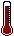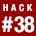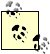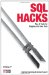# Hack 38. Find Transposition ErrorsWhen you have a list of numbers and a target batch total to check it against, you can often spot a transposition error and narrow down the probable source.

Consider how travel expenses are processed. Say that every item you receive is accompanied by a paper receipt and is entered into a database. When you add the values on the receipts using a calculator, you get 133.56, but the database says the total is 131.76.

In Table 5-20, the "Correct values" column shows the numbers as they appear on paper; the "Incorrect values" column shows what has been entered into the database. Notice that the values are in pence or cents. Having a decimal point makes little difference, but it is neater to work with whole numbers. The challenge is to track down the transposition error where the value 1754 has been entered as 1574.

Table 5-20. Expense reconciliation

Correct values Incorrect values (the column v)
2460 2460
1452 1452
1450 1450
1610 1610
1772 1772
1160 1160
**Transposition error** 175 4 157 4
1698 1698
Total 13356 13176

The difference in the totals is 180. The rule of thumb is this: when a batch totals error is a multiple of 9 the problem is likely to be a transposition error. In this case, the magnitude of the difference tells us that the transposition is between the hundreds column and the tens column (giving us 18). Further, because 18 / 9 = 2, you know that the digit difference is 2, and you are looking for 02, 13, 24, 35, 46, 57, 68, or 79 in those two columns of v. In this example, the column v total is lower than the correct total. If the total in column v had been higher than the other total you would be looking for 20, 31, 42, and so on.If the two-digit number ab is transposed to ba the difference is divisible by 9: (10x a + b) (10x b + a) = 9x (a b). This property of being divisible by 9 is preserved even when the pairs occur in larger numbers, as long as no other errors are made.

The magnitude of the error gives you the column position and the digit difference helps find the pattern required. You can calculate these values using numbers as numbers or numbers as strings:

```mysql> SET @correctTotal = 13356;
Query OK, 0 rows affected (0.00 sec)

mysql> SET @diff = (SELECT SUM(v) FROM incorrect) - @correctTotal;
Query OK, 0 rows affected (0.00 sec)

mysql> SET @magnitude = POWER(10,FLOOR(LN(ABS(@diff))/LN(10))-1);
Query OK, 0 rows affected (0.00 sec)
mysql> SET @digitDiff = @diff/@magnitude/9;
Query OK, 0 rows affected (0.00 sec)

mysql> SELECT @diff, @magnitude, @digitDiff;
+---------+------------+------------+
| @diff | @magnitude | @digitDiff |
+---------+------------+------------+
| -180.00 | 10 | -2 |
+---------+------------+------------+```I've used the term magnitude here to mean the largest power of 10 that divides the difference. So the magnitude of 180 is 10 and the magnitude of 27,000 is 1,000. The expression LN(x) / LN(10) is the logarithm of x base 10; if you take the FLOOR of that value you've got the number of digits. You use the POWER function to turn that digit count back into a number of the right magnitude.

Using the magnitude, you can extract the two candidate digits from each number in the list. This query extracts the relevant pair of digits from each number in the batch, and it displays the digit difference:

```mysql> SELECT v,FLOOR(v/@magnitude/10) % 10 AS digitA,
-> FLOOR(v/@magnitude) % 10 AS digitB,
-> FLOOR(v/@magnitude/10) % 10 - FLOOR(v/@magnitude) % 10
->  AS digitDiff
-> FROM incorrect;
+---------+--------+--------+-----------+
| v | digitA | digitB | digitDiff |
+---------+--------+--------+-----------+
| 2460.00 | 4 | 6 | -2 |
| 1452.00 | 4 | 5 | -1 |
| 1450.00 | 4 | 5 | -1 |
| 1610.00 | 6 | 1 | 5 |
| 1772.00 | 7 | 7 | 0 |
| 1160.00 | 1 | 6 | -5 |
| 1574.00 | 5 | 7 | -2 |
| 1698.00 | 6 | 9 | -3 |
+---------+--------+--------+-----------+```

You are looking for a digit difference of 2 between columns digitA and digitB; if these columns are switched the result would account for the 180 error in the batch total. For example, if you switched the 4 and the 6 in the number 2,460 the result would be 180 more, and that's exactly what you need to get your totals to agree.

```mysql> SELECT
-> v,
-> CASE WHEN FLOOR(v/@magnitude/10) % 10 -
-> FLOOR(v/@magnitude) % 10=@digitDiff THEN '*******'
-> ELSE '' END
-> AS investigate
-> FROM incorrect;
+---------+-------------+
| v | investigate |
+---------+-------------+
| 2460.00 | ******* |
| 1452.00 | |
| 1450.00 | |
| 1610.00 | |
| 1772.00 | |
| 1160.00 | |
| 1574.00 | ******* |
| 1698.00 | |
+---------+-------------+```

You cannot tell which of the two marked values is responsible for the error; in fact, you cannot be certain that it was a transposition that caused the error. However, this tells you where to start your investigation.

5.15.1. SQL Server

The same code runs more or less unchanged in SQL Server. However, you must declare your variable as INTEGER and the logarithm function is called LOG, not LN:

```DECLARE @correctTotal AS INTEGER
DECLARE @diff AS INTEGER
DECLARE @magnitude AS INTEGER
DECLARE @digitDiff AS INTEGER
SET @correctTotal = 13356
SET @diff = (SELECT SUM(v) FROM incorrect) - @correctTotal
SET @magnitude = POWER(10,FLOOR(LOG(ABS(@diff))/LOG(10))-1)
SET @digitDiff = @diff/@magnitude/9
SELECT @correctTotal, @diff, @magnitude, @digitDiff;
SELECT v,FLOOR(v/@magnitude/10) % 10 x,FLOOR(v/@magnitude) % 10 y
FROM incorrect
SELECT
v,
CASE WHEN FLOOR(v/@magnitude/10) % 10-
FLOOR(v/@magnitude) % 10=@digitDiff THEN '*******' END
investigate
FROM incorrect;
GO```

5.15.2. Oracle

In Oracle, the method for setting and retrieving variables is slightly different. You can declare variables and assign them using the SELECT ... INTO statement from inside a BEGIN END block.

You use the MOD function in place of the % operator:

```set serveroutput on
DECLARE
correctTotal NUMBER := 13356;
diff NUMBER;
magnitude NUMBER;
digitDiff NUMBER;
v NUMBER;
id NUMBER;
CURSOR mCursor IS
SELECT t.v, 10 * t.x + t.y - (10 * t.y + t.x) id
FROM
(SELECT v, MOD(FLOOR(v/magnitude/10),10) x,
MOD(FLOOR(v/magnitude), 10) y
FROM incorrect) t
WHERE 9*(x-y) = diff/magnitude;
a NUMBER;
b NUMBER;
BEGIN
SELECT SUM(v)- correctTotal
INTO diff
FROM incorrect;
SELECT POWER(10,FLOOR(LN(ABS(diff))/LN(10))-1)
INTO magnitude
FROM dual;
SELECT diff/magnitude/9
INTO digitDiff
FROM dual;
dbms_output.put_line(correctTotal);
dbms_output.put_line(diff);
dbms_output.put_line(magnitude);
dbms_output.put_line(digitDiff);

OPEN mCursor;
LOOP
FETCH mCUrsor INTO a,b;
EXIT WHEN mCUrsor%NOTFOUND;
dbms_output.put_line(a);
dbms_output.put_line(b);
END LOOP;
CLOSE mCursor;
END;
/```

5.15.3. Single Query

Each example shown so far uses programming constructs, but these are really for convenience, they are not essential. You can run the entire process as a single SQL statement:

```mysql> SELECT v,
-> CASE WHEN FLOOR(v/magnitude/10) % 10 -
-> FLOOR(v/magnitude) % 10=digitDiff
-> THEN '*******'
-> ELSE '' END AS investigate
-> FROM incorrect CROSS JOIN
-> (SELECT batchTot, diff, magnitude, digitDiff
-> FROM
-> (SELECT
-> diff/magnitude/9 AS digitDiff,
-> magnitude,diff,batchTot
-> FROM
-> (SELECT
-> POWER(10,FLOOR(LN(ABS(diff))/LN(10))-1)
-> AS magnitude,
-> diff,batchTot
-> FROM
-> (SELECT batchTot-13356 AS diff, batchTot
-> FROM
-> (SELECT SUM(v) AS batchTot FROM incorrect)
-> t1) t2) t3) t4) t5
-> ;
+---------+-------------+
| v | investigate |
+---------+-------------+
| 2460.00 | ******* |
| 1452.00 | |
| 1450.00 | |
| 1610.00 | |
| 1772.00 | |
| 1160.00 | |
| 1574.00 | ******* |
| 1698.00 | |
+---------+-------------+```

You need to make some minor changes to get this to work on other platforms:

1. SQL Server uses LOG rather than LN.
2. Oracle uses MOD(FLOOR(v/magnitude/10),10) rather than the % operator.
3. In PostgreSQL, you must use CAST(diff/magnitude/9) AS digitDiff.SQL Hacks
ISBN: 0596527993
EAN: 2147483647
Year: 2004
Pages: 147